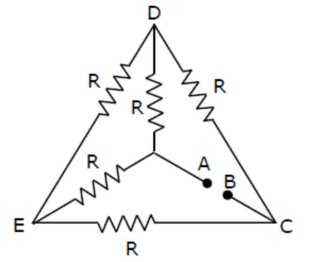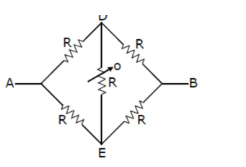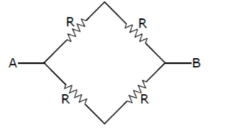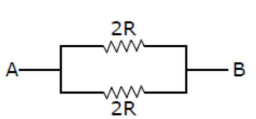# Five equal resistances are connected in a network as shown in figure.Question:

Five equal resistances are connected in a network as shown in figure. The net resistance between the points $A$ and $B$ is :1. (1) $\frac{3 R}{2}$

2. (2) $\frac{\mathrm{R}}{2}$

3. (3) $\mathrm{R}$

4. (4) $2 \mathrm{R}$

Correct Option: , 3

Solution:

$(3)$

It is balanced wheat stone bridgeSo, we know that

$\mathrm{R}_{1} \mathrm{R}_{\mathrm{A}}=\mathrm{R}_{2} \mathrm{R}_{3}$

$\frac{\mathrm{R}_{1}}{\mathrm{R}_{2}}=\frac{\mathrm{R}_{3}}{\mathrm{R}_{4}}$$\operatorname{Req}=\frac{2 R \times 2 R}{2 R+2 R}$

$\frac{4 R^{2}}{4 R} \Rightarrow R$